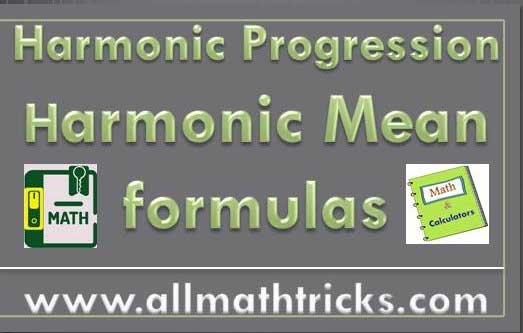# Harmonic Progression Formula, Properties and Harmonic Mean Formula

In this article we learn about harmonic progression formula for nth term, sum of terms, properties with examples.
Harmonic mean formula for two quantities, three quantities and number of quantities.

## Harmonic Progression and Harmonic Mean formulas with properties

Definition of Harmonic Progression

A series of terms is known as a Harmonic progression series when the reciprocals of elements are in arithmetic progression.

In general, If p, q, r, s are in arithmetic progression then 1/p, 1/q, 1/r,  1/s are all in Harmonic progression.

For example take the series 1/10, 1/12, 1/14, 1/16 . . . . . . . .

Here reciprocal of the given series is 10, 12, 14, 16 . . . . . . .

The series is clearly in Arithmetic progression having first term is ’10’ and common difference is ‘2’

So the given series is in Harmonic progression

Harmonic progression nth term & sum formula

In order find the nth term or sum of terms in a Harmonic Progression, one should make the series into corresponding arithmetic series and then find nth term of the series.

Let 1/a, 1/(a+d), 1/(a + 2d), . . . . . . is in an HP then the inverse of a harmonic  progression follows the rule of an arithmetic progression. So

a, a + d, a + 2d, . . . . . . .  are in AP and first term is ‘a’  ,  common difference is “d”

nth term of A.P  an = a + (n -1) d

So nth term of given HP isSimilarly sum of ‘n’ terms of AP is  Sn

Sum of  ‘n’ terms of given HP isNote: The sum of a harmonic series will never be an integer except first term is “1” and number of terms are  “1”.

Properties of Harmonic Progression

1. Any number of quantities are said to be in harmonic progression when every three consecutive terms are in harmonic progression.

2. Three quantities p, q , r  are said to be Harmonic Progression

So 1/p, 1/q , 1/r  are in arithmetic progression

Common difference of the series  d  = 1/q – 1/p = 1/r – 1/q⇒3. Selection of terms in Harmonic Progression

 Number of terms Terms 3 1/(a–d), 1/a, 1/(a+d) 4 1/(a–3d), 1/(a–d), 1/(a+d), 1/(a+3d) 5 1/(a–2d), 1/(a–d), 1/a, 1/(a+d), 1/(a+2d)

### Harmonic mean between given quantities

Harmonic mean between two quantities

Let p, q be the two quantities and H is a harmonic mean of their quantities. Then 1/p, 1/H and 1/q are in Arithmetic progression

Therefore

⇒⇒i.e if p, q & r be the three quantities are in harmonic progression  then is a harmonic mean of their quantities.

Therefore, harmonic mean formula

Harmonic meanHarmonic mean between number of quantities

If r1, r2, r3, r4, . . . . .  rn be an finite “n” non-zero numbers  are in HP. Then harmonic mean “H” of these numbers is given by

1/H  = (1/n) [ 1/ r1 + 1/r2 + 1/r3 + 1/r4,+. . . . .  +1/ rn ]Related Articles

Sequence and series

Arithmetic Progression Formulas

Arithmetic Progression Questions with Solutions

Arithmetic Mean formula with Examples

Geometric Progression formulas

Geometric Progression Examples

Harmonic Progression formulas

Relation between AM, GM and HM